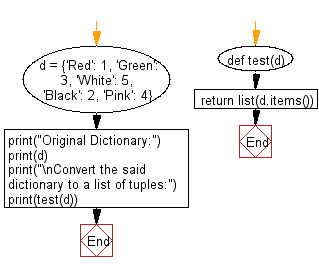﻿ Python: Convert a given dictionary to a list of tuples - w3resource# Python: Convert a given dictionary to a list of tuples

## Python dictionary: Exercise-77 with Solution

Write a Python program to convert given a dictionary to a list of tuples.

• Use dict.items() and list() to get a list of tuples from the given dictionary.

Sample Solution:

Python Code:

``````def test(d):
return list(d.items())

d = {'Red': 1, 'Green': 3, 'White': 5, 'Black': 2, 'Pink': 4}
print("Original Dictionary:")
print(d)
print("\nConvert the said dictionary to a list of tuples:")
print(test(d))
```
```

Sample Output:

```Original Dictionary:
{'Red': 1, 'Green': 3, 'White': 5, 'Black': 2, 'Pink': 4}

Convert the said dictionary to a list of tuples:
[('Red', 1), ('Green', 3), ('White', 5), ('Black', 2), ('Pink', 4)]
```

Flowchart:## Visualize Python code execution:

The following tool visualize what the computer is doing step-by-step as it executes the said program:

Python Code Editor:

Have another way to solve this solution? Contribute your code (and comments) through Disqus.

What is the difficulty level of this exercise?

Test your Programming skills with w3resource's quiz.

﻿

## Python: Tips of the Day

Decapitalizes the first letter of a string:

Example:

```def tips_decapitalize(s, upper_rest=False):
return s[:1].lower() + (s[1:].upper() if upper_rest else s[1:])
print(tips_decapitalize('PythonTips'))
print(tips_decapitalize('PythonTips', True))
```

Output:

```pythonTips
pYTHONTIPS
```